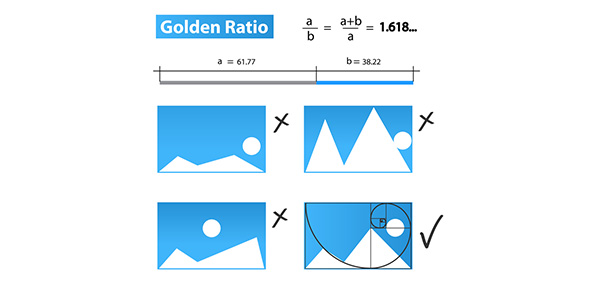# Factors, Ratios And Percents

4 QuestionsSettingsRelated Topics
• 1.
At a dinner party each table can seat eight people. If 100 people attend the party, what is the minimum number of tables that are needed?
• A.

12

• B.

13

• C.

14

• D.

15

• E.

16

• 2.
Let x, y, and z be positive integers such that y is a multiple of x and z. All of the following statements are true except for:
• A.

Y.z is a multiple of x

• B.

X is a factor of y

• C.

X.z is a factor of y

• D.

Z divides evenly into y

• E.

X divides evenly into y.z

• 3.
When a two-digit number is divided by 5 the remainder is 2. Which of the following statements must be true about the two-digit number?
• A.

The sum of all the digits is odd.

• B.

The digit in the one’s place is odd.

• C.

The number is prime.

• D.

The number is odd.

• E.

The digit in the one’s place is prime.

• 4.
When a two-digit number is divided by 5 the remainder is 2. Which of the following statements must be true about the two-digit number?
• A.

The sum of all the digits is odd.

• B.

The digit in the one’s place is odd.

• C.

The number is prime.

• D.

The number is odd.

• E.

The digit in the one’s place is prime.